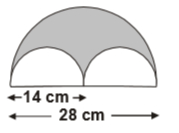# User Forum

Subject :IMO    Class : Class 7

In the given figure, the area (in square centimetres) of the shaded portion isA308
B462
C154
D150

Class : Class 9

Class : Class 7
option a

## Ans 3:

Class : Class 7
yes it should be 154

## Ans 4:

Class : Class 8
the correct answer is B. there are two small semicircles that form one circle when joined. so after joining it forms 1 circle with a diameter of 14 which means it has a radius of 7. area= 7*7*(22/7)= 154 in the big semicircle it has a diameter of 28 which means it has a radius of 14. area = (14*14*22)/(7*2) = 616 area of shaded part = 616-154= 462 thus the correct option is B